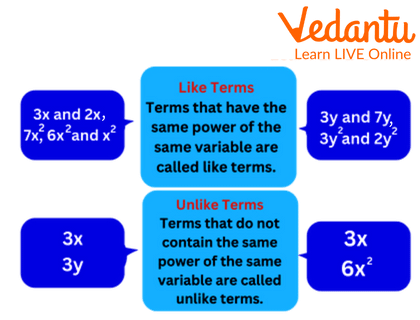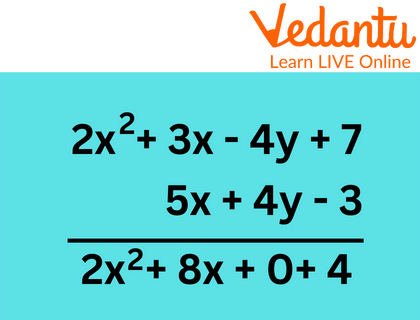Courses
Courses for Kids
Free study material
Free LIVE classes
More## Introduction

The addition of algebraic expressions and the addition of numbers are very similar operations. The classification of terms in an algebraic expression into similar and unlike terms is necessary for the addition of algebraic expressions, then picking up and including similar terms. Similar to this, only the numerical coefficient can be adjusted.Like terms and Unlike Terms

## How to Add Algebraic Expressions:

In order to do addition of algebraic expressions, we must first collect all similar terms. The only like term whose coefficient is the combination of the coefficients of like terms is the sum of the like terms.

Will three pencils and three erasers work? NO will be your answer. Three pencils and three erasers are two different objects; hence we can't add them together. The situation with terms in an algebraic expression is identical. No more than two opposite terms can be combined. It's important to keep in mind that we can only add similar terms to algebraic expressions. Algebraic expressions sums can be obtained by using one of two methods:

1. Horizontal method of Algebra Addition

2. Column method for Algebra Addition

## Horizontal Method of Algebra Addition

The following list of steps outlines how to add algebraic expressions using the horizontal method:

Step1: Write all the expressions on a horizontal line between an additional sign and brackets.

Step 2: Rewrite the expression after collecting all the similar terms from all the expressions.

Step 3: Add the numerical coefficients of all the similar terms and the common variable.

Step 4: Rewrite the phrase to make it more concise, and ensure that all of the terms in the final solution are opposite terms.

## Column Method of Algebra Addition

Step1: Write each expression one below the other in step one. Make sure all terms are in the same column.

$\begin{array}{l}2{x^2} + 3x - 4y + 7\\{\rm{ }}5x + 4y - 3\end{array}$

For instance, if a term appears in the first expression but its equivalent does not appear in the second, either write that term below it or leave that column empty.

Step 2: Write the common variable in the same column after adding the numerical coefficients of each column (similar terms).Column Method

Step 3: Rewrite the final response, $2{x^2} + 8x + 4$

## Conclusion

We need to first assemble all related terms before we can add algebraic expressions. The sum of similar terms is the only like term whose coefficient combines the coefficients of other like terms.

## Solved Examples

Example 1: What equation should $[3{a^2} - 5b + 2c]$ be subtracted from to arrive at $\left( {{a^2} + 5c} \right)$ as the solution?

Ans: We must sum both formulas $[3{a^2} - 5b + 2c)$+$\left[{{a^2} + 5c} \right]$ $= 4{a^2} - 5b + 7c$ to determine from $[3{a^2} - 5b + 2c]$what should be subtracted to get $\left[ {{a^2} + 5c} \right]$.

$[3{a^2} - 5b + 2c]$ should be subtracted from $= 4{a^2} - 5b + 7c$

Example 2: Add $6x + 4y - 7z,3x - 2y,x + 8y - 9z$

Ans: Writing like terms one below the other , we will get:

+$\begin{array}{l}6x + 4y - 7z \\3x - 2y \\x + 8y - 9z\end{array}$

$10x + 10y - 16z$

$10x + 10y - 16z$

Example 3: 5. Add the $\left[ {3x + 2y} \right]$ and $\left( {x + y} \right]$ by horizontal method.
Ans: Horizontal Method:
$\left( {3x + 2y} \right] + \left[ {x + y} \right)$
Arrange the like terms together, and then add.
$\begin{array}{l} = 3x + 2y + x + y\\ = 4x + 3y\end{array}$

Last updated date: 02nd Jun 2023
Total views: 46.8k
Views today: 0.42k

1. What Are the Two Fundamental Principles for the Solution of Algebraic Expressions?

Only like terms can be added or removed when solving algebraic formulas. It's important to resolve the brackets first.

2. How Can You Simplify and Combine Like Terms?

Group all related terms together, add or remove the related terms' numerical coefficients, and then add the common variable to it.

3. How Do You Add unlike Terms?

Unlike terms cannot be combined.

4. In mathematics, what are Like terms?

The phrases that have the same variable raised to the same power are referred to as similar terms in algebra.

5. How are three algebraic expressions added together?

Write the given expressions in rows and arrange them in the same order. Similar terms should be noted down below one another and added in columns. Sort the expressions provided. Similar terms should be noted down below one another and added in columns.